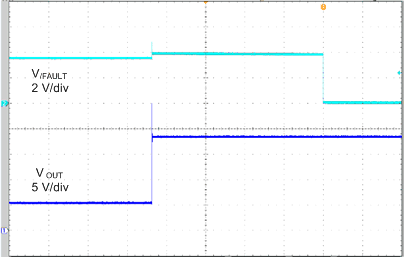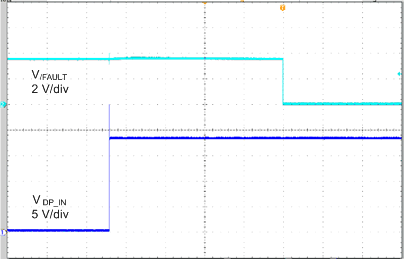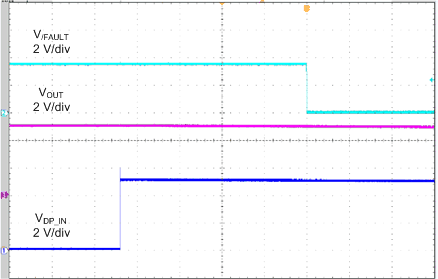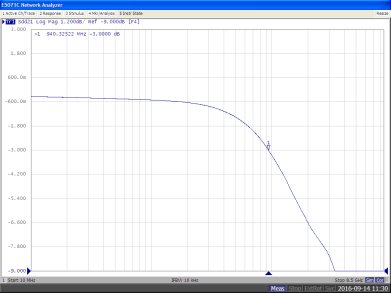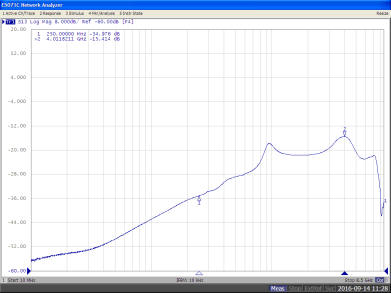JAJSCJ4A September   2016  – October 2016

PRODUCTION DATA.

1. 特長
2. アプリケーション
3. 概要
4. 改訂履歴
5. Pin Configuration and Functions
6. Specifications
7. Parameter Measurement Information
8. Detailed Description
1. 8.1 Overview
2. 8.2 Functional Block Diagram
3. 8.3 Feature Description
4. 8.4 Device Functional Modes
9. Application and Implementation
1. 9.1 Application Information
2. 9.2 Typical Application
1. 9.2.1 Design Requirements
2. 9.2.2 Detailed Design Procedure
3. 9.2.3 Application Curves
10. 10Power Supply Recommendations
11. 11Layout
12. 12デバイスおよびドキュメントのサポート
1. 12.1 デバイス・サポート
2. 12.2 ドキュメントのサポート
3. 12.3 ドキュメントの更新通知を受け取る方法
4. 12.4 コミュニティ・リソース
5. 12.5 商標
6. 12.6 静電気放電に関する注意事項
7. 12.7 Glossary
13. 13メカニカル、パッケージ、および注文情報

• RVC|20
• RVC|20

## 6 Specifications

### 6.1 Absolute Maximum Ratings

Voltages are with respect to GND unless otherwise noted(1)
MIN MAX UNIT
Voltage range CS, CTL1, CTL2, EN, FAULT, ILIM_HI, ILIM_LO, IN, IMON, OVP_SEL, STATUS –0.3 7 V
DM_OUT, DP_OUT –0.3 5.7
BIAS, DM_IN, DP_IN, OUT –0.3 18
Continuous current DM_IN to DM_OUT or DP_IN to DP_OUT –100 100 mA
OUT Internally limited
ISRC Continuous output source current ILIM_HI, ILIM_LO, IMON Internally limited A
ISNK Continuous output sink current FAULT, STATUS 25 mA
CS Internally limited A
TJ Operating junction temperature –40 Internally limited °C
Tstg Storage temperature –65 150 °C
Stresses beyond those listed under Absolute Maximum Ratings may cause permanent damage to the device. These are stress ratings only, which do not imply functional operation of the device at these or any other conditions beyond those indicated under Recommended Operating Conditions. Exposure to absolute-maximum-rated conditions for extended periods may affect device reliability.

### 6.2 ESD Ratings

VALUE UNIT
V(ESD) Electrostatic discharge Human-body model (HBM), per AEC Q100-002(1) ±2 000(2) V
Charged-device model (CDM), per AEC Q100-011 ±750(3)
IEC 61000-4-2 contact discharge, DP_IN and DM_IN(4) ±8 000
IEC 61000-4-2 air discharge, DP_IN and DM_IN(4) ±15 000
AEC Q100-002 indicates that HBM stressing shall be in accordance with the ANSI/ESDA/JEDEC JS-001 specification.
The passing level per AEC-Q100 Classification H2.
The passing level per AEC-Q100 Classification C5
Surges per IEC 61000-4-2, level 4, 1999 applied from DP_IN and DM_IN to output ground of the TPS254900Q1EVM-817 (SLUUBI0) evaluation module.

### 6.3 Recommended Operating Conditions

Voltages are with respect to GND unless otherwise noted.
MIN NOM MAX UNIT
V(IN) Supply voltage IN 4.5 6.5 V
Input voltage CTL1, CTL2, EN, OVP_SEL 0 6.5 V
DM_IN, DM_OUT, DP_IN, DP_OUT 0 3.6 V
I(OUT) Output continuous current OUT (–40°C ≤ TA ≤ 85°C) 3 A
DM_IN to DM_OUT or DP_IN to DP_OUT –30 30 mA
Continuous output sink current FAULT, STATUS 10 mA
R(ILIM_xx) Current-limit-set resistors 14.3 1000
TJ Operating junction temperature –40 125 °C

### 6.4 Thermal Information

THERMAL METRIC(1) TPS254900-Q1 UNIT
RVC (WQFN)
16 PINS
RθJA Junction-to-ambient thermal resistance 37.9 °C/W
RθJC(top) Junction-to-case (top) thermal resistance 39.9 °C/W
RθJB Junction-to-board thermal resistance 11.9 °C/W
ψJT Junction-to-top characterization parameter 0.5 °C/W
ψJB Junction-to-board characterization parameter 11.8 °C/W
RθJC(bot) Junction-to-case (bottom) thermal resistance 3.2 °C/W

### 6.5 Electrical Characteristics

Unless otherwise noted, –40°C ≤ TJ ≤ 125°C and 4.5 V ≤ V(IN) ≤ 6.5 V, V(EN) = V(CTL1) = V(CTL2) = V(IN), R(FAULT) = R(STATUS) = 10 kΩ, R(IMON) = 2.55 kΩ, R(ILIM_HI) = 19.1 kΩ, R(ILIM_LO) = 80.6 kΩ. Positive currents are into pins. Typical values are at 25°C. All voltages are with respect to GND.
PARAMETER TEST CONDITIONS MIN TYP MAX UNIT
OUT – POWER SWITCH
rDS(on) On-resistance(1) TJ = 25°C 45 55
–40°C ≤ TJ ≤ 85°C 45 69
–40°C ≤TJ ≤ 125°C 45 77
Ilkg Reverse leakage current VOUT = 6.5 V, VIN = VEN = 0 V, –40°C ≤ TJ ≤ 85°C, measure I(IN) 0.01 2 µA
OUT – DISCHARGE
R(DCHG) Discharge resistance (mode change) 400 500 630 Ω
CTL1, CTL2, EN, OVP_SEL INPUTS
Input pin rising logic threshold voltage 1 1.35 2 V
Input pin falling logic threshold voltage 0.85 1.15 1.65 V
Hysteresis(2) 200 mV
Input current Pin voltage = 0 V or 6.5 V –1 1 µA
CURRENT LIMIT
IOS OUT short-circuit current limit R(ILIM_LO) = 210 kΩ 190 240 290 mA
R(ILIM_LO) = 80.6 kΩ 555 620 680
R(ILIM_LO) = 21.5 kΩ 2145 2300 2460
R(ILIM_LO) = 19.1 kΩ 2420 2590 2760
R(ILIM_HI) = 18.2 kΩ 2545 2720 2895
R(ILIM_HI) = 14.3 kΩ 3240 3455 3670
R(ILIM_HI) shorted to GND 5000 6500 8000
SUPPLY CURRENT
I(IN_OFF) Disabled IN supply current V(EN) = 0 V, V(OUT) = 0 V, –40°C ≤ TJ ≤ 85°C, no 5.1-kΩ resistor (open) between BIAS and OUT 0.1 5 µA
I(IN_ON) Enabled IN supply current SDP mode (CTL1, CTL2 = 0, 1) 170 250 µA
CDP mode (CTL1, CTL2 = 1, 1) 200 280
Client mode (CTL1, CTL2 = 0, 0) 120 210
UNDERVOLTAGE LOCKOUT, IN
V(UVLO) UVLO threshold voltage IN rising 3.9 4.15 4.3 V
Hysteresis(3) TJ = 25°C 100 mV
FAULT
Output low voltage I(FAULT) = 1 mA 100 mV
Off-state leakage V(FAULT) = 6.5 V 2 µA
STATUS
Output low voltage I(STATUS) = 1 mA 100 mV
Off-state leakage V(STATUS) = 6.5 V 2 µA
THERMAL SHUTDOWN
T(OTSD2) Thermal shutdown threshold 155 °C
T(OTSD1) Thermal shutdown threshold in current-limit 135 °C
Hysteresis(3) 20 °C
LOAD DETECT (VCTL1 = VCTL2 = VIN)
I(LD) IOUT load detection threshold R(ILIM_LO) = 80.6 kΩ, rising load current 585 650 715 mA
Hysteresis(3) 50 mA
DM_IN AND DP_IN OVERVOLTAGE PROTECTION
V(OV_Data) Protection trip threshold DP_IN and DM_IN rising 3.7 3.9 4.15 V
Hysteresis(3) 100 mV
R(DCHG_Data) Discharge resistor after OVP(2) DP_IN = DM_IN = 18 V, IN = 5 V or 0 V 200
DP_IN = DM_IN = 5 V, IN = 5 V 370
DP_IN = DM_IN = 5 V, IN = 0 390
OUT OVERVOLTAGE PROTECTION
V(OV_OUT_LOW) Protection trip threshold OUT rising 5.65 6 6.35 V
Hysteresis(3) 90 mV
V(OV_OUT_HIGH) Protection trip threshold OUT rising 6.6 6.95 7.3 V
Hysteresis(3) 130 mV
R(DCHG_OUT) Discharge resistor OUT = 18 V, IN = 5 V 55 85
OUT = 18 V, IN = 0 80 120
CABLE COMPENSATION
I(CS) Sink current Load = 3 A, 2.5 V ≤ V(CS) ≤ 6.5 V 234 246 258 µA
Load = 2.4 A, 2.5 V ≤ V(CS) ≤ 6.5 V 187 197 207
Load = 2.1 A, 2.5 V ≤ V(CS) ≤ 6.5 V 163 172 181
Load = 1 A, 2.5 V ≤ V(CS) ≤ 6.5 V 77 82 87
CURRENT MONITOR OUTPUT (IMON)
I(IMON) Source current Load = 3 A, 0 ≤ V(IMON) ≤ 2.5 V 287 312 337 µA
Load = 2.4 A, 0 ≤ V(IMON) ≤ 2.5 V 230 250 270
Load = 2.1 A, 0 ≤ V(IMON) ≤ 2.5 V 201 218 235
Load = 1 A, 0 ≤ V(IMON) ≤ 2.5 V 94 104 114
Load = 0.5 A, 0 ≤ V(IMON) ≤ 2.5 V 44 52 60
HIGH-BANDWIDTH ANALOG SWITCH
R(HS_ON) DP and DM switch on-resistance V(DP_OUT) = V(DM_OUT) = 0 V, I(DP_IN) = I(DM_IN) = 30 mA 3.2 6.5 Ω
V(DP_OUT) = V(DM_OUT) = 2.4 V, I(DP_IN) = I(DM_IN) = –15 mA 3.8 7.6
|ΔR(HS_ON)| Switch resistance mismatch between DP and DM channels V(DP_OUT) = V(DM_OUT) = 0 V, I(DP_IN) = I(DM_IN) = 30 mA 0.05 0.15 Ω
V(DP_OUT) = V(DM_OUT) = 2.4 V, I(DP_IN) = I(DM_IN) = –15 mA 0.05 0.15
C(IO_OFF) DP and DM switch off-state capacitance(4) VEN = 0 V, V(DP_IN) = V(DM_IN) = 0.3 V, Vac = 0.03 VPP , f = 1 MHz 8.8 pF
C(IO_ON) DP and DM switch on-state capacitance(4) V(DP_IN) = V(DM_IN) = 0.3 V, Vac = 0.03 VPP, f = 1 MHz 10.9 pF
Off-state isolation(3) V(EN) = 0 V, f = 250 MHz 8 dB
On-state cross-channel isolation(4) f = 250 MHz 30 dB
Ilkg(OFF) Off-state leakage current VEN = 0 V, V(DP_IN) = V (DM_IN) = 3.6 V, V(DP_OUT) = V(DM_OUT) = 0 V, measure I(DP_OUT) and I(DM_OUT) 0.1 1.5 µA
BW Bandwidth (–3 dB)(4) R(L) = 50 Ω 940 MHz
CHARGING DOWNSTREAM PORT DETECT
V(DM_SRC) DM_IN CDP output voltage V(DP_IN) = 0.6 V, –250 µA < I(DM_IN) < 0 µA 0.5 0.6 0.7 V
V(DAT_REF) DP_IN rising lower window threshold for V(DM_SRC) activation 0.36 0.4 V
Hysteresis(4) 50 mV
V(LGC_SRC) DP_IN rising upper window threshold for VDM_SRC de-activation 0.8 0.88 V
V(LGC_SRC_HYS) Hysteresis(4) 100 mV
I(DP_SINK) DP_IN sink current V(DP_IN) = 0.6 V 40 75 100 µA
Pulse-testing techniques maintain junction temperature close to ambient temperature. Thermal effects must be taken into account separately.
This parameter is provided for reference only and does not constitute part of TI's published device specifications for purposes of TI's product warranty.
This parameter is provided for reference only and does not constitute part of TI's published device specifications for purposes of TI's product warranty.
This parameter is provided for reference only and does not constitute part of TI's published device specifications for purposes of TI's product warranty.

### 6.6 Switching Characteristics

Unless otherwise noted –40°C ≤ TJ ≤ 125°C and 4.5 V ≤ V(IN) ≤ 6.5 V, V(EN) = V(IN), V(CTL1) = V(CTL2) = V(IN). R(FAULT) = R(STATUS) = 10 kΩ, R(IMON) = 2.55 KΩ, R(ILIM_HI) = 19.1 kΩ, R(ILIM_LO) = 80.6 kΩ. Positive currents are into pins. Typical values are at 25°C. All voltages are with respect to GND.
PARAMETER TEST CONDITIONS MIN TYP MAX UNIT
tr OUT voltage rise time V(IN) = 5 V, C(L) = 1 µF, R(L) = 100 Ω 1.05 1.75 3.1 ms
tf OUT voltage fall time 0.27 0.47 0.82 ms
ton OUT voltage turnon time V(IN) = 5 V, C(L) = 1 µF, R(L) = 100 Ω 7.5 11 ms
toff OUT voltage turnoff time 2.7 5 ms
t(DCHG_S) Discharge hold time (mode change) Time V(OUT) < 0.7 V 1.1 2 2.9 s
t(IOS) OUT short-circuit response time(1) V(IN) = 5 V, R(SHORT) = 50 mΩ 2 µs
t(OC_OUT_FAULT) OUT FAULT deglitch time Bidirectional deglitch applicable to current-limit condition only (no deglitch assertion for OTSD) 5.5 8.5 11.5 ms
tpd Analog switch propagation delay (1) V(IN) = 5 V 0.14 ns
t(SK) Analog switch skew between opposite transitions of the same port (tPHL – tPLH) (1) V(IN) = 5 V 0.02 ns
t(LD_SET) Load-detect set time V(IN) = 5 V 120 210 280 ms
t(LD_RESET) Load-detect reset time V(IN) = 5 V 1.8 3 4.2 s
t(OV_Data) DP_IN and DM_IN overvoltage protection response time 5 µs
t(OV_OUT) OUT overvoltage protection response time 0.3 µs
t(OV_D_FAULT) DP_IN and DM_IN FAULT-asserted degltich time 11 16 23 ms
OUT FAULT-asserted degltich time 11 16 23 ms
These parameters are provided for reference only and do not constitute part of TI's published device specifications for purposes of TI's product warranty.

### 6.7 Typical Characteristics

TA = 25°C, V(IN) = 5 V, V(EN) = 5 V, V(CTL1) = V(CTL2) = 5 V, FAULT and STATUS connect to V(IN) via a 10-kΩ pullup resistor (unless stated otherwise)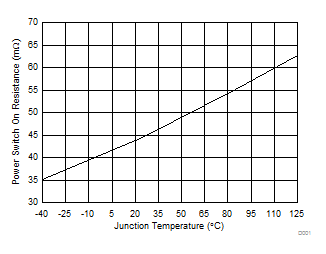V(IN) = 5 V
Figure 1. Power Switch On-Resistance vs Temperature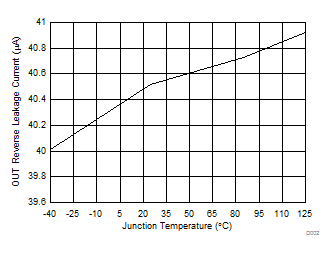V(OUT) = 5 V Measure I(OUT)
Figure 2. Reverse Leakage Current vs TemperatureA
Figure 3. OUT Discharge Resistance (Mode Change) vs Temperature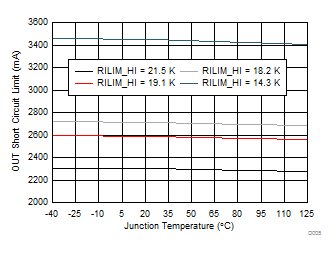V(IN) = 5 V
Figure 5. OUT Short-Circuit Current Limit vs Temperature I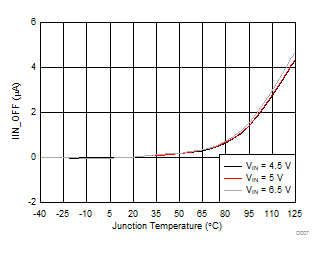CTL1 = 1 CTL2 = 1
Figure 7. Disabled IN Supply Current vs Temperature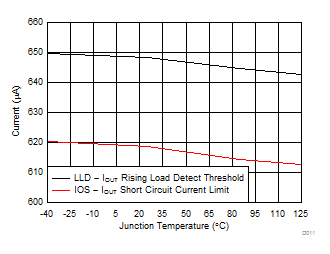V(IN) = 5 V R(ILIM_LO) = 80.6 kΩ
Figure 9. I(OUT) Rising Load-Detect Threshold and OUT Short-Circuit Limit vs Temperature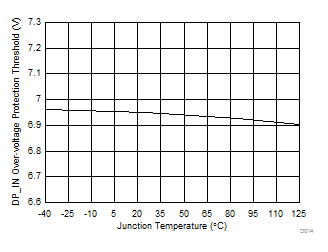V(IN) = 5 V
Figure 11. OUT Overvoltage Protection Threshold vs Temperature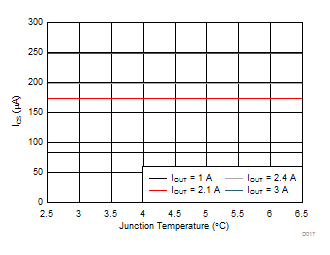VIN = 6.5 V
Figure 13. I(CS) vs V(CS) Voltage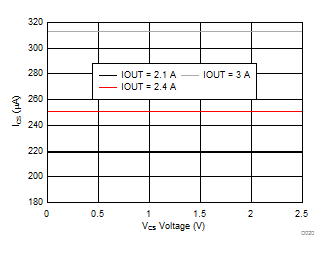VIN = 4.5 V
Figure 15. I(IMON) vs V(CS) Voltage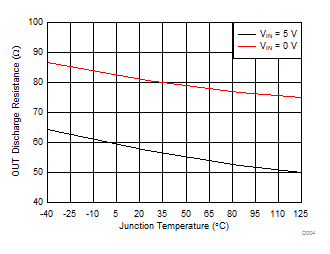A
Figure 4. OUT Discharge Resistance (OVP) vs Temperature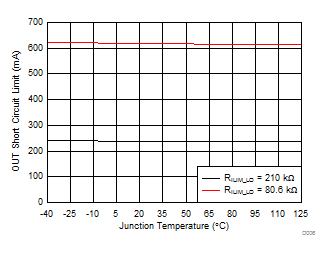V(IN) = 5 V
Figure 6. OUT Short-Circuit Current Limit vs Temperature II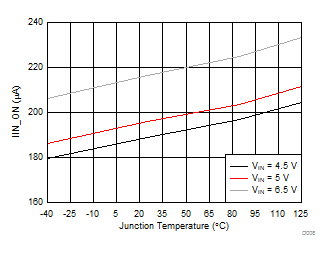CTL1 = 1 CTL2 = 1
Figure 8. Enabled IN Supply Current – CDP (11) vs Temperature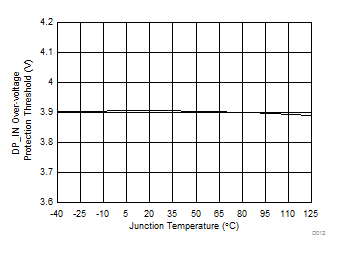V(IN) = 5 V
Figure 10. DP_IN Overvoltage Protection Threshold vs TemperatureV(IN) = 5 V V(CS) = 25 V
Figure 12. I(CS) vs Temperature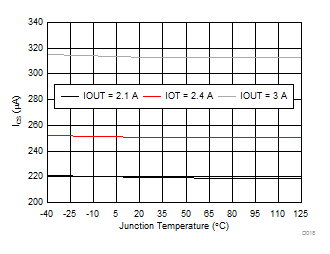VIN = 5 V V(IMON) = 25 V
Figure 14. I(IMON) vs Temperature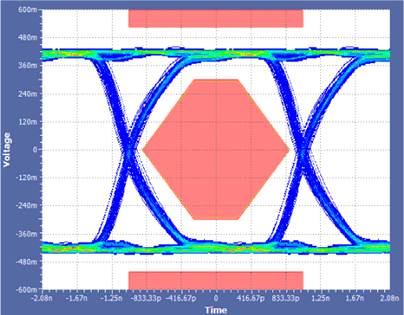Measured on EVM with 10-cm cable
Figure 16. Bypassing the TPS254900-Q1 Data SwitchR(LOAD) = 5 Ω C(LOAD) = 10 µF t = 2 ms/div
Figure 18. Turnon Response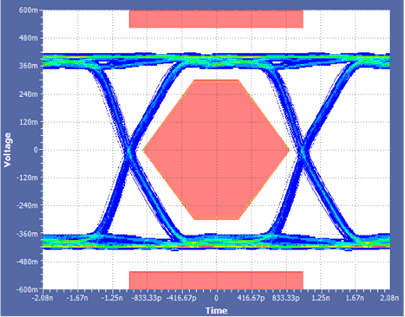Measured on EVM with 10-cm cable
Figure 17. Through the TPS254900-Q1 Data Switch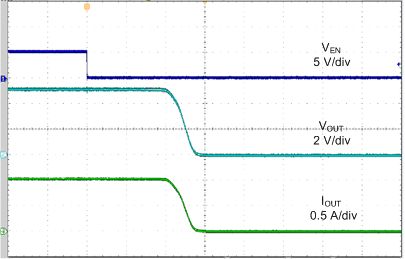R(LOAD) = 5 Ω C(LOAD) = 10 µF t = 1 ms/div
Figure 19. Turnoff ResponseR(ILIM_LO) = 80.6 kΩ t = 4 ms/div
Figure 20. Enable Into Short (SDP)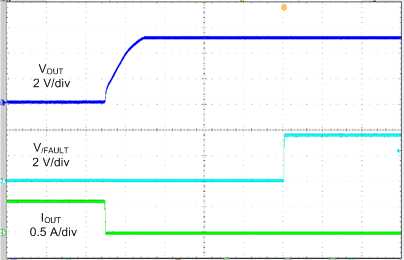R(ILIM_LO) = 80.6 kΩ t = 2 ms/div
Figure 22. Short Circuit to No Load (SDP)R(ILIM_HI) = 19.1 kΩ R(short) = 50 mΩ t = 2 ms/div
Figure 24. Hot Short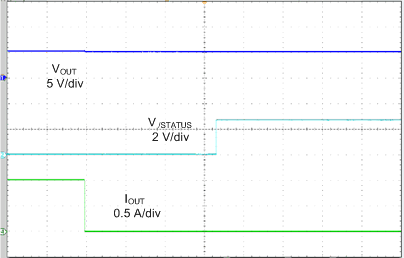R(ILIM_LO) = 80.6 kΩ t = 1 s/div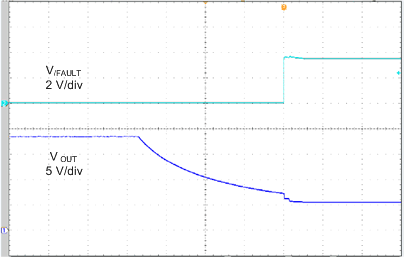t = 100 ms/div
Figure 28. OUT Short-to-Battery Recovery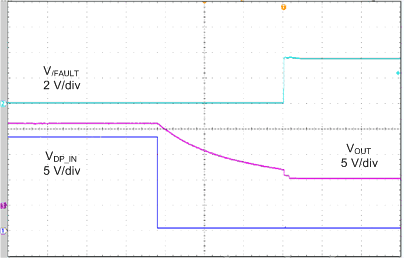R(BIAS) = 5.1 kΩ t = 100 ms/div
Figure 30. DP_IN Short-to-Battery Recovery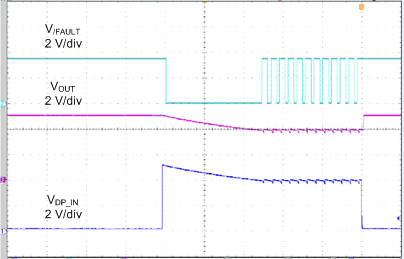R(BIAS) = 5.1 kΩ t = 200 ms/div
Figure 32. DP_IN Short-to-VBUS and Recovery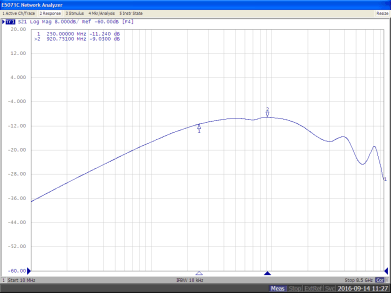Figure 34. Off-State Data-Switch Isolation vs Frequency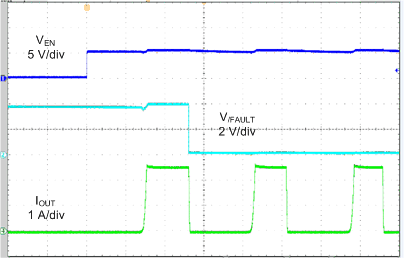R(ILIM_HI) = 19.1 kΩ t = 4 ms/div
Figure 21. Enable Into Short (CDP) – Thermal Cycling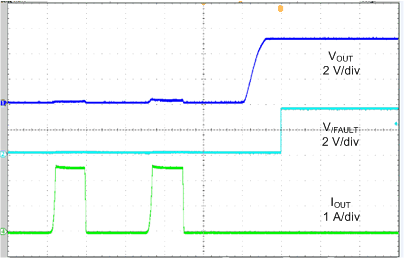R(ILIM_HI) = 19.1 kΩ t = 4 ms/div
Figure 23. Short Circuit to No Load (CDP)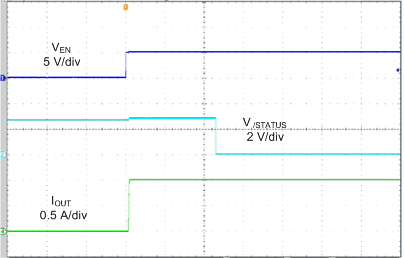R(ILIM_LO) = 80.6 kΩ t = 100 ms/div